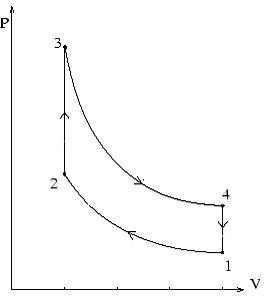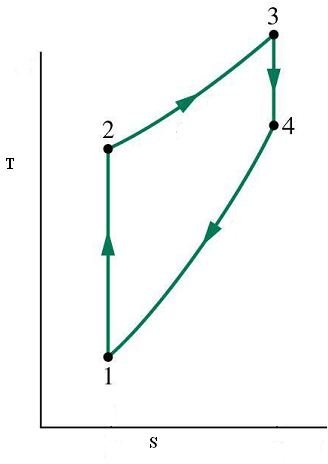# The Otto Cycle in Marine Diesel Engines: Articles on Theoretical Cycles

## Introduction

Internal combustion engines are those where the combustion of the fuel-air mixture takes place within a closed chamber known as the combustion chamber. They work mostly on one of the three cycles namely Otto cycle, Diesel cycle or Dual cycle. Since diesel engines are also internal combustion engines do not jump to the conclusion that diesel engines only operate on the diesel cycle simply because of the name of the cycle. Diesel engines can operate on any on these cycles and we will study these cycles one by one. Do keep one thing in mind while studying these cycles that no actual engine strictly follows these cycles, still they are a useful tool to compare the performance of actual engines under different conditions

## The Otto Cycle

This cycle is also known by the name of constant volume cycle and it consists of the following four steps. Please take a thorough look at the P-V and T-S diagrams depicting these processes along with reading the description and you will understand it in a better way. These diagrams are given below this section. Also remember that though in a real engine it is not pure air which is worked up but in these cycles air is considered as the working substance.

• 1-2 process is that of isentropic compression of air which means that the entropy of the air remains constant during this transition and can be seen from figure 2 (T-S diagram). It can also be seen from the same diagram that temperature of the air rises during this process, while the volume decreases significantly and pressures rises (figure 1).
• 2-3 process represents addition of heat to the air at a constant volume which results in increase of entropy, temperature and pressure of the air
• 3-4 is the isentropic expansion of air which corresponds to the power stroke of the engine where the pressure generated during combustion is used to drive the piston upwards and hence deliver motion to the outside system. The temperature of the air falls in this process and so does the pressure, and volume returns to the original volume to what it was at point 1.
• 4-1 is the process of heat rejection at constant volume which ultimately brings the working substance or air back to the initial condition. In an actual cycle there is the exhaust cycle at this stage but here no expulsion of the working substance is assumed.

Since thermal efficiency of a cycle represents the heat converted into work per unit of heat added the thermal efficiency of an Otto cycle is given by

Thermal Efficiency = 1-(T4 – T1)/(T3 – T2)

Using the relationships of perfect Gas laws which I am omitting here to avoid complexity the thermal efficiency can also be written as

Thermal Efficiency = 1 – (1/r)^(k-1)

Where r is compression ratio and k is a constant depending on the properties of the working substance.

## P-V and T-S Diagrams for Otto Cycle